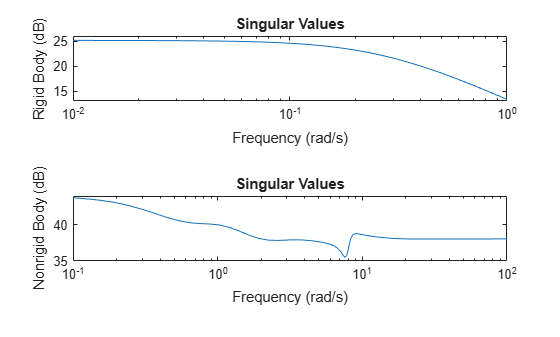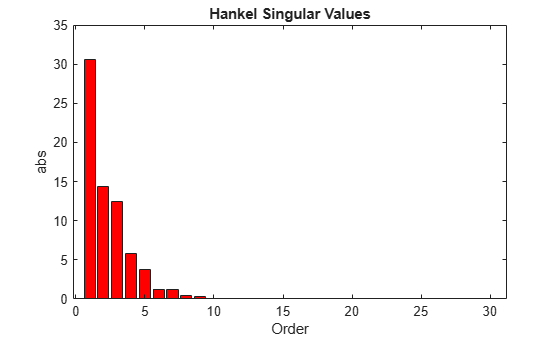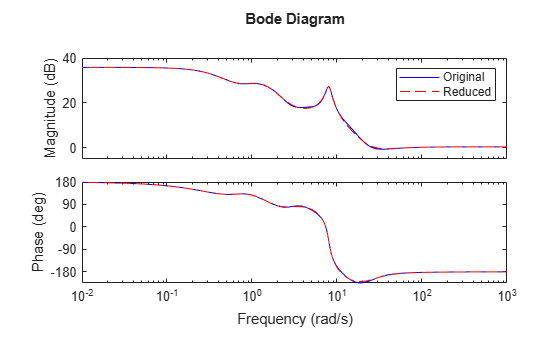Documentation

## Using Modal Algorithms

### Rigid Body Dynamics

In many cases, a model's $j\omega$-axis poles are important to keep after model reduction, e.g., rigid body dynamics of a flexible structure plant or integrators of a controller. A unique routine, `modreal`, serves the purpose nicely.

`modreal` puts a system into its modal form, with eigenvalues appearing on the diagonal of its A-matrix. Real eigenvalues appear in 1-by-1 blocks, and complex eigenvalues appear in 2-by-2 real blocks. All the blocks are ordered in ascending order, based on their eigenvalue magnitudes, by default, or descending order, based on their real parts. Therefore, specifying the number of $j\omega$-axis poles splits the model into two systems with one containing only $j\omega$-axis dynamics, the other containing the remaining dynamics.

```rng(5678,'twister'); G = rss(30,1,1); % random 30-state model [Gjw,G2] = modreal(G,1); % only one rigid body dynamics G2.D = Gjw.D; % put DC gain of G into G2 Gjw.D = 0; subplot(2,1,1) sigma(Gjw) ylabel('Rigid Body') subplot(2,1,2) sigma(G2) ylabel('Nonrigid Body')```Further model reduction can be done on `G2` without any numerical difficulty. After `G2` is further reduced to `Gred`, the final approximation of the model is simply `Gjw+Gred`.

This process of splitting $j\omega$-axis poles has been built in and automated in all the model reduction routines `balancmr`, `schurmr`, `hankelmr`, `bstmr`, and `hankelsv`, so that users need not worry about splitting the model.

Examine the Hankel singular value plot.

`hankelsv(G)`Calculate an eighth-order reduced model.

```[gr,info] = reduce(G,8); figure bode(G,'b-',gr,'r--') legend('Original','Reduced')```The default algorithm `balancmr` of `reduce` has done a great job of approximating a 30-state model with just eight states. Again, the rigid body dynamics are preserved for further controller design.

## Related Topics

##### Support평가판 신청